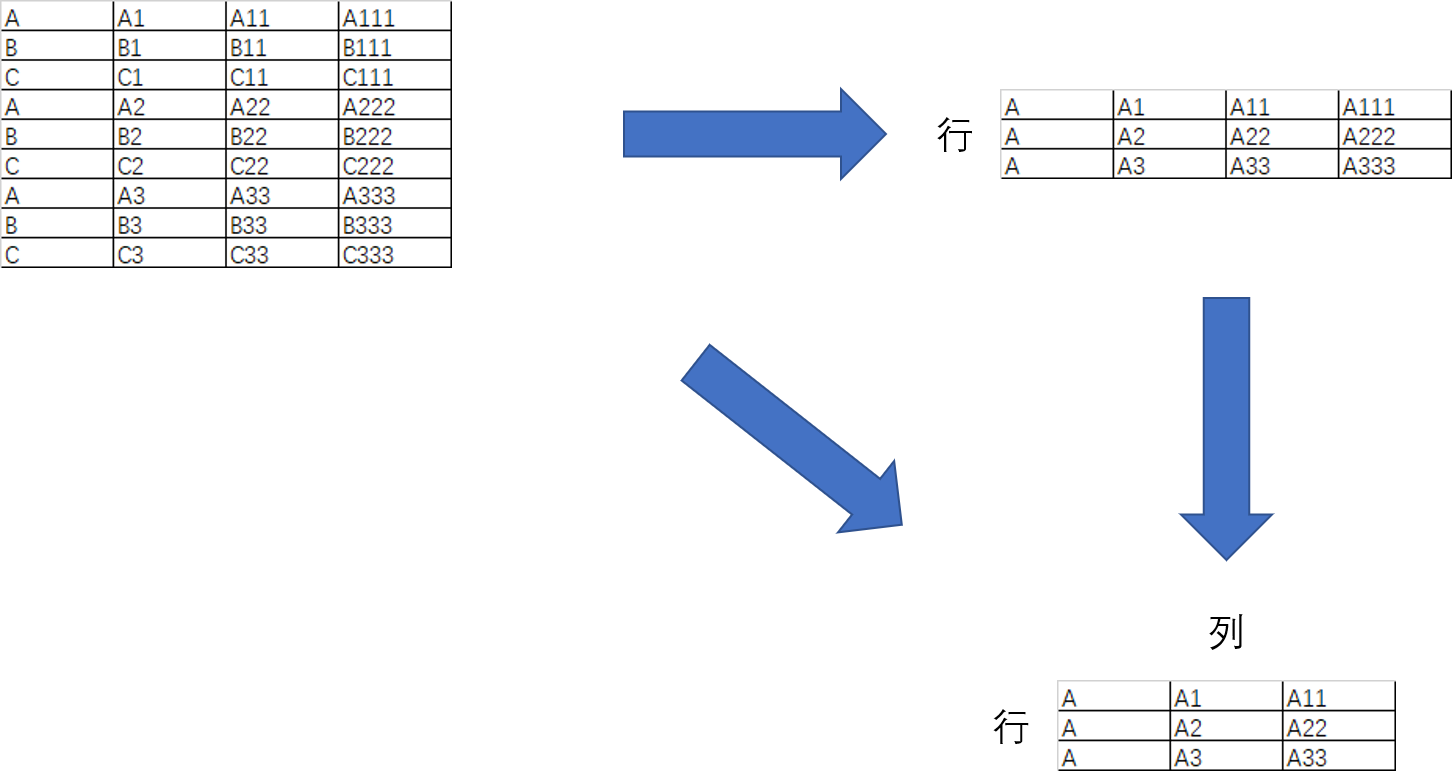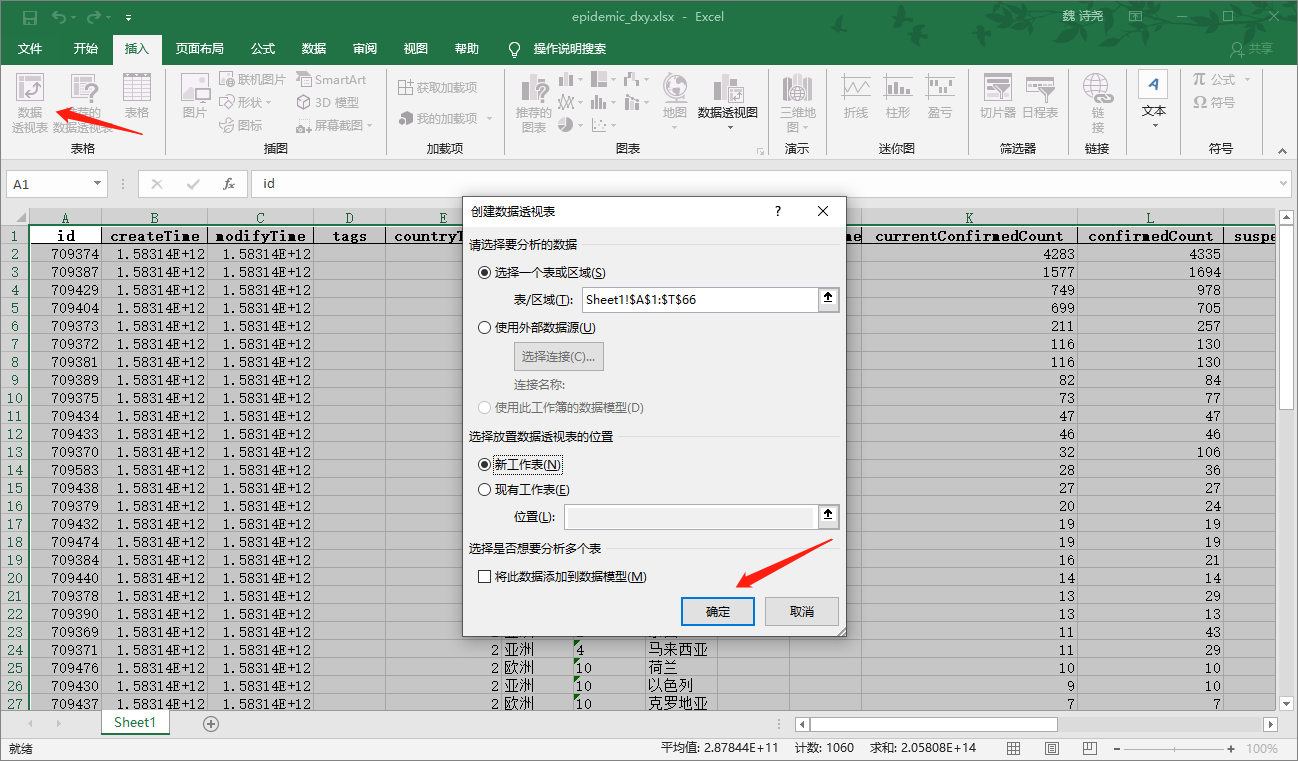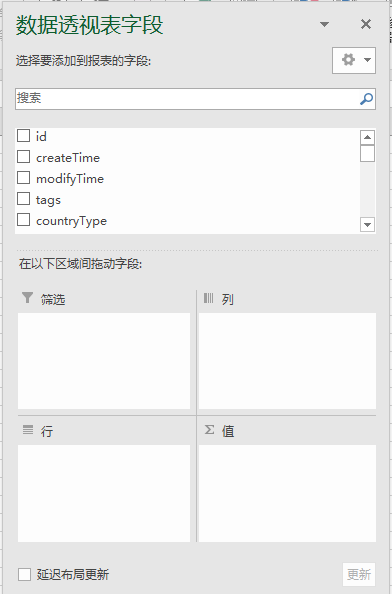# 小白学 Python 数据分析（12）：Pandas （十一）数据透视表（pivot_table）

2019/04/10 10:10## 数据透视表pandas.pivot_table(data, values=None, index=None, columns=None, aggfunc='mean', fill_value=None, margins=False, dropna=True, margins_name='All', observed=False) → 'DataFrame'

• data: 需要做数据透视的整个表
• values: 要汇总的数据项
• index: 在数据透视表索引上进行分组的键
• columns: 在数据透视表列上进行分组的键
• aggfunc: 对 values 的计算类型
• fill_value: 空值的填充值
• margins: 是否显示合计
• dropna: 是否删除缺失，如果未是，则删除缺失数据的那一行
• margins_name: 合计类的列名

import pandas as pd

# 数据导入

df = pd.pivot_table(epidemic_dxy, values='currentConfirmedCount', index='continents', aggfunc='sum')

print(df)

# 输出内容
currentConfirmedCount
continents



import pandas as pd

# 数据导入

df1 = pd.pivot_table(epidemic_dxy, values='currentConfirmedCount', index='continents', columns='provinceName', aggfunc='sum')

print(df1)

# 输出内容
provinceName   丹麦  亚美尼亚  以色列   伊拉克     伊朗  ...   阿曼   阿联酋      韩国  马来西亚  黎巴嫩
continents                                 ...

[7 rows x 65 columns]


import pandas as pd

# 数据导入

df2 = pd.pivot_table(epidemic_dxy, values='currentConfirmedCount', index=['continents', 'createTime'], columns='provinceName', aggfunc='sum')

print(df2)

# 输出内容
provinceName               丹麦  亚美尼亚  以色列   伊拉克  ...   阿联酋      韩国  马来西亚  黎巴嫩
continents createTime                           ...

1583138991000  NaN   1.0  NaN   NaN  ...   NaN     NaN   NaN  NaN

1583138991000  NaN   NaN  NaN   NaN  ...   NaN     NaN   NaN  NaN

1583138991000  NaN   NaN  NaN   NaN  ...   NaN     NaN   NaN  NaN

1583138991000  NaN   NaN  NaN   NaN  ...   NaN     NaN   NaN  NaN

[11 rows x 65 columns]


## 示例代码

0
0 收藏

### 作者的其它热门文章0 评论
0 收藏
0